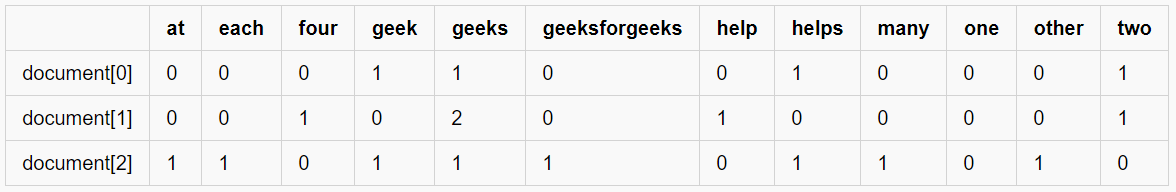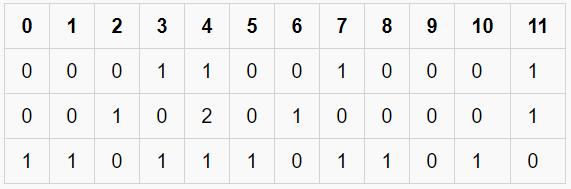Related Articles

# Using CountVectorizer to Extracting Features from Text

• Difficulty Level : Basic
• Last Updated : 17 Jul, 2020

CountVectorizer is a great tool provided by the scikit-learn library in Python. It is used to transform a given text into a vector on the basis of the frequency (count) of each word that occurs in the entire text. This is helpful when we have multiple such texts, and we wish to convert each word in each text into vectors (for using in further text analysis).

Let us consider a few sample texts from a document (each as a list element):

document = [ “One Geek helps Two Geeks”, “Two Geeks help Four Geeks”, “Each Geek helps many other Geeks at GeeksforGeeks.”]

CountVectorizer creates a matrix in which each unique word is represented by a column of the matrix, and each text sample from the document is a row in the matrix. The value of each cell is nothing but the count of the word in that particular text sample.

This can be visualized as follows –Key Observations:

1. There are 12 unique words in the document, represented as columns of the table.
2. There are 3 text samples in the document, each represented as rows of the table.
3. Every cell contains a number, that represents the count of the word in that particular text.
4. All words have been converted to lowercase.
5. The words in columns have been arranged alphabetically.

Inside CountVectorizer, these words are not stored as strings. Rather, they are given a particular index value. In this case, ‘at’ would have index 0, ‘each’ would have index 1, ‘four’ would have index 2 and so on. The actual representation has been shown in the table below –A Sparse Matrix

This way of representation is known as a Sparse Matrix.

Code: Python implementation of CountVectorizer

 `from` `sklearn.feature_extraction.text ``import` `CountVectorizer`` ` `document ``=` `[``"One Geek helps Two Geeks"``,``            ``"Two Geeks help Four Geeks"``,``            ``"Each Geek helps many other Geeks at GeeksforGeeks"``]`` ` `# Create a Vectorizer Object``vectorizer ``=` `CountVectorizer()`` ` `vectorizer.fit(document)`` ` `# Printing the identified Unique words along with their indices``print``(``"Vocabulary: "``, vectorizer.vocabulary_)`` ` `# Encode the Document``vector ``=` `vectorizer.transform(document)`` ` `# Summarizing the Encoded Texts``print``(``"Encoded Document is:"``)``print``(vector.toarray())`

Output:

```Vocabulary:  {'one': 9, 'geek': 3, 'helps': 7, 'two': 11, 'geeks': 4, 'help': 6, 'four': 2, 'each': 1, 'many': 8, 'other': 10, 'at': 0, 'geeksforgeeks': 5}

Encoded Document is:

[ [0 0 0 1 1 0 0 1 0 1 0 1]

[0 0 1 0 2 0 1 0 0 0 0 1]

[1 1 0 1 1 1 0 1 1 0 1 0] ]```

Attention reader! Don’t stop learning now. Get hold of all the important Machine Learning Concepts with the Machine Learning Foundation Course at a student-friendly price and become industry ready.

My Personal Notes arrow_drop_up